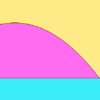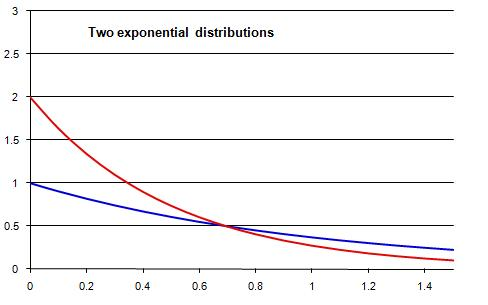#### You may also like### PDF

Given a probability density function find the mean, median and mode of the distribution.### Scale Invariance

By exploring the concept of scale invariance, find the probability that a random piece of real data begins with a 1.### PCDF

When can a pdf and a cdf coincide?

# Into the Exponential Distribution

##### Age 16 to 18Challenge LevelThis chart shows the probability density functions for two exponential distributions. Here are some questions to consider concerning these sorts of distributions. You can use a mixture of probability-based reasoning and algebraic reasoning based on the properties of integrals and areas.

1. Which exponential distributions do these curves correspond to? What interesting mathematical properties do they have? Which has the largest mean?
2. Two separate areas are enclosed by the red and blue curves: one to the left of the point of intersection and one to the right. What can you say about the sizes of these two areas? Can you give a good explanation?
3. Can you find the point of intersection of the pdfs? Can you find the area of each of the enclosed parts of the diagrams?
4. From the graphs, I visually estimated that $P(0.5 < Red < 0.7) = 0.125$. Can you work out how I made this estimate? Is it an over-estimate or an underestimate? How close do you think that estimate is to the correct answer? Work out the correct answer to find out.
5. Try to make, assess and check your own estimates similar to that in question 4.